## Tuesday, June 30, 2009

### 484

484 = 22 x 112

484 is a square number: 22 x 22 = 484.

484 is a palindrome in base 3 (12221) and in base 10.

484 has exactly nine divisors: 1, 2, 4, 11, 22, 44, 121, 242, 484.Juliett 484 was a Soviet guided-missile submarine that eventually ended up on display at the Russian Sub Museum in Providence, R.I.

Source: The Positive Integers

## Monday, June 29, 2009

### 382

382 = 2 x 191.

382 is the maximum number of regions into which 20 circles divide the plane.

382 is the sum of ten consecutive primes: 19 + 23 + 29 + 31 + 37 + 41 + 43 + 47 + 53 + 59 = 382.

382 is a Smith number.

The sum of the divisors of 382 is a square: 1 + 2 + 191 + 382 = 576 = 242.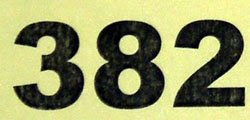382 Dodona is a large main-belt asteroid discovered in 1894.

Source:
Wikipedia

## Friday, June 26, 2009

### 256

256 = 28. It is the smallest prime power of a prime power of a prime power of a prime.

256 is a square number: 16 x 16 = 256.

256 is the number of possible values that can be stored in a byte (8 bits).

256 is one of two fourth powers using the same digits: 44 = 256 and 54 = 625.

256 is the number of integer partitions of 29 into distinct parts.256 is the number of drams in a pound.

Source:
Numeropedia

## Thursday, June 25, 2009

### 150

150 = 2 x 3 x 52.

2
150 – 3 and 2150 – 5 are twin primes.

150 is the largest gap between consecutive twin prime pairs less than a thousand. It occurs between {659, 661} and {809, 811}.

Each internal angle of a regular 12-sided polygon (dodecagon) is
150o.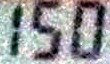Sesquicentennial is a period of
150 years.

Source:
Prime Curios!

## Wednesday, June 24, 2009

### 37

37 is a prime number.

37 is the least number of 5th powers needed to represent every possible integer.

37 is the maximum number of regions into which 8 lines divide the plane.

The sum of the first
37 primes is a Fibonacci number.

37 has a representation as a sum of two squares: 37 = 12 + 62.

37 is the hypotenuse of a primitive Pythagorean triple: 372 = 122 + 352.

37 is a centered hexagonal number.Abraham Lincoln was first elected to the U.S. House of Representatives at age
37.

Source:
37 Heaven!

## Tuesday, June 23, 2009

### 1123

1123 is a prime number.

1123 is a prime of the form n2 + n + 1, for n = 33.

1123 has digits that start the Fibonacci sequence.

1123 is the smallest prime showing three consecutive digits.

1123 is a divisor of 333 - 1.11/23 (November 23) is the only date containing the concatenation of two 2-digit primes that can be read as a prime number in either "month-day" or "day-month" format, i.e., 11/23 or 23/11.

## Monday, June 22, 2009

### 995

995 = 5 x 199.

995 is a square-free number because its prime decomposition contains no repeated factors.

995 is the smallest multiple of 5 whose digits sum to 23.

995 is a number that can't be expressed as the sum of a prime number and a Fibonacci number.995 is the approximate speed (in meters per second) of Mach 3 (three times the speed of sound).

Source: Number Gossip

## Friday, June 19, 2009

### 888

888 = 23 x 3 x 37.

888 is the only number whose cube (700227072) consists of three digits each occurring three times.

888 and the following 18 integers are all composite numbers.

888 is 4040 in base 6.

888 is the sum of eight consecutive primes: 97 + 101 + 103 + 107 + 109 + 113 + 127 + 131 = 888.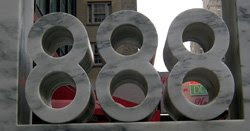888 is the number to key in for the letter “T” on a telephone keypad when text messaging.

Source: Number Gossip

## Thursday, June 18, 2009

### 769

769 is a prime number.

769 is the total number of digits of all binary numbers of length 1 to 7.

769 is 30001 in base 4.

769 has a representation as a sum of two squares: 769 = 122 + 252.

769 is the hypotenuse of a primitive Pythagorean triple: 7692 = 4812 + 6002.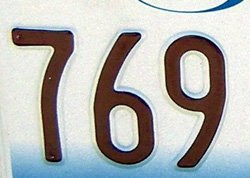769 Tatjana is an asteroid orbiting the sun.

## Wednesday, June 17, 2009

### 650

650 = 2 x 52 x 13.

650 is a pronic number. It is the product of two consecutive integers: 25 x 26 = 650.

650 is the sum of the first 12 squares. Hence, it is a pyramidal number.

650 is 22022 in base 4 and 1616 in base 7.In the 1950s, advanced features of the IBM 650 Magnetic Drum Data Processing Machine brought increased reliability to the young field of electronic computing.

Source: Number Gossip

## Tuesday, June 16, 2009

### 518

518 = 2 x 7 x 37.

518 = 51 + 12 + 83.

518 is 2222 in base 6.

518 is a number n such that n2 has the property that the sum of its digits and the product of its digits are nonzero squares: 5182 = 268324; 2 + 6 + 8 + 3 + 2 + 4 = 25 = 252; 2 x 6 x 8 x 3 x 2 x 4 = 2304 = 482.518 is the telephone area code for Albany, N.Y.

## Monday, June 15, 2009

### 466

466 = 2 x 233. It is a semiprime that is the product of two distinct Sophie Germain primes.

Each digit of 466 is a semiprime.

466 is 1234 in base 7.

466 has a representation as a sum of two squares: 466 = 52 + 212.

466 arises in the sequence created by starting with 1 and repeatedly reversing the digits and adding five: 1, 6, 11, 16, 66, 71, . . . , 164, 466, 669, . . .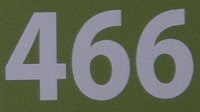466 is the maximum number of regions into which 30 lines divide a plane.

Source: Positive Integers

## Friday, June 12, 2009

### 346

346 = 2 x 173.

346 is a Smith number. The sum of its digits equals the sum of all the digits appearing in its prime divisors (excluding 1).

346 is a Franel number.

346 has a representation as a sum of two squares: 346 = 112 + 152.The
M-346 is an advanced fighter trainer aircraft being developed in Italy.

Source:
Number Gossip

## Thursday, June 11, 2009

### 237

237 = 3 x 79.

237 is a number n such that n2 + 2 is a prime: 2372 + 2 = 56171.

237 is the smallest three-digit number with its digits being three distinct primes that is itself not prime and none of the permutations of its digits represents a prime number.

237 is the smallest number with the property that its first three multiples contain the digit 7: 237, 474, and 711.Room
237 plays a significant role in the Stanley Kubrick film The Shining.

Source:
Prime Curios!

## Wednesday, June 10, 2009

### 131

131 is a prime number. It is a Sophie Germain prime because 2 x 131 + 1 = 263 is also prime.

The
131st Fibonacci number (1066340417491710595814572169) is the smallest Fibonacci prime that contains all the digits from 0 to 9.

131 is the smallest prime that stays prime when the end digits (on both sides) are repeated once: 11311 is also prime.

131 is the smallest integer such that the sum of its digits in every base b = 2, 3, 4, 5, 6, 7, 8, 9, 10, 11 is prime.Locomotive
131 plunges off the edge of a ravine in the movie Back to the Future Part III.

Source:
Prime Curios!

## Tuesday, June 9, 2009

### 35

35 = 5 x 7.

35 is the sum of the cubes of the first two primes: 23 + 33 = 35.

The product of
35 and 36 has the same prime factors as the product of 4374 and 4375.

35 can be written as a sum of odd primes in 35 ways, and as a sum of primes in 35 x 5 ways.

35 has a unique representation as a sum of three squares: 35 = 12 + 32 + 52.

35 is the smallest number with five representations as a sum of three distinct primes: 35 = 3 + 13 + 19 = 5 + 7 + 23 = 5 + 11 + 19 = 5 + 13 + 17 = 7 + 11 + 17.

35 is the fifth tetrahedral number (the sum of the first five triangular numbers).

35 is the fourth pentatope number.

35 is 55 in base 6.There are
35 hexominoes, polyominoes made from six squares.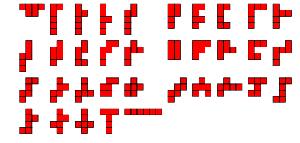Source:
Number Gossip

## Monday, June 8, 2009

### 1004

1004 = 22 x 251.

1004 is a heptanacci number.

1004 is a number n such that the sum of its divisors is a square: 1 + 2 + 2 + 251 = 256 = 162.

1004 is the sum of five positive fifth powers: 1004 = 35 + 35 + 35 + 35 + 25.

1004 is number n such that 2n + 1 is a semiprime: 2009 = 72 x 41.In the Star Wars saga, CC-
1004 was a senior clone commander in the Grand Army of the Republic.

Source:
On-Line Encyclopedia of Integer Sequences

## Friday, June 5, 2009

### 908

908 = 22 x 227.

908 can be expressed as 67 + 179 + 283 + 379. When any three of these primes are added together, the result is a perfect square. 908 is the smallest sum of four primes that has this property.

908 is an even number that is not the sum of two primes, each of which is one of a pair of twin primes.

908 is the smallest of three consecutive integers divisible by three consecutive primes respectively: 908 is divisible by 2, 909 is divisible by 3, and 910 is divisible by 5.908 is the telephone area code for New Brunswick, N.J.

Source: Prime Curios!

## Thursday, June 4, 2009

### 851

851 = 23 x 37.

851 is the numbered of ordered partitions of 18 into distinct parts.

851 is 3535 in base 6.

851 is a sum of fifth powers of primes.The Ducati 851 was a motorcycle first available to the public in 1987.

## Wednesday, June 3, 2009

### 780

780 = 22 x 3 x 5 x 13.

780 is a triangular number, the sum of the positive integers from 1 to 39.

780 = (5 + 7) × (5 + 8) × (5 + 0).

780 is the sum of ten consecutive primes: 59 + 61 + 67 + 71 + 73 + 79 + 83 + 89 + 97 + 101 = 780.Telephone area code 780 covers Edmonton, Alberta.

## Tuesday, June 2, 2009

### 665

665 = 5 x 7 x 19.

665 is the only odd multiple of 5 that is expressible as the difference of sixth powers of two consecutive (2 and 3) primes: 36 – 26 = 665.

665 is 555 in base 11.

665 is a divisor of 113 - 1.The Indian mathematician and astronomer Brahmagupta (born 598) died in the year 665.

Source: Prime Curios!

## Monday, June 1, 2009

### 543

543 = 3 x 181.

543 is a number whose square (5432 = 294,849) and cube (5433 = 160,103,007) use different digits.

543 is a number that cannot be written as the sum of three squares.

543 is a number n such that n2 has the property that the sum of its digits and the product of its digits are nonzero squares: 5432 = 294849; 2 + 9 + 4 + 8 + 4 + 9 = 36 = 362; 2 x 9 x 4 x 8 x 4 x 9 = 20736 = 1442.543 Charlotte is an asteroid discovered in 1904.